Geometry, unlike the other mathematical subjects tested on the GRE, has special rules. Basically, these rules prohibit test-takers from just eye-balling the answers based off of the diagrams. So for example, in the following problem:

Example 1
Find the value of: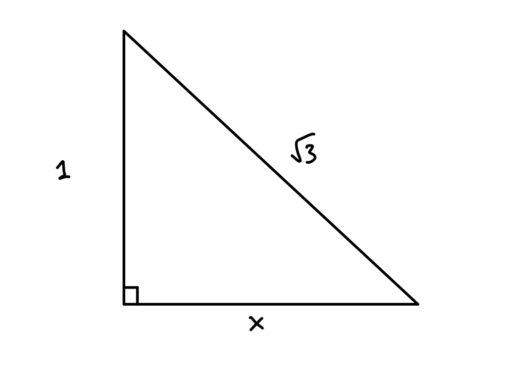You cannot just look at the figure and guess that the mystery leg is the same length as the other leg of the triangle. For if we used the Pythagorean Theorem to calculate the solution:

So our first rule is:

Rule 1: Shapes and figures are not necessarily drawn to scale.

So in the following problem:

Example 2
Is side B longer than side A?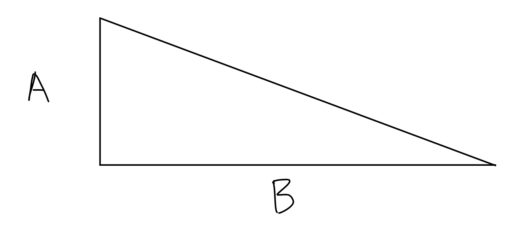The correct answer is that it cannot be determined which side is longer.

However, this does not mean that the figures drawn are totally misleading. For we also have:

Rule 2: Shapes and figures do show the relative position of different objects.

What does this mean? It means that if you see something like: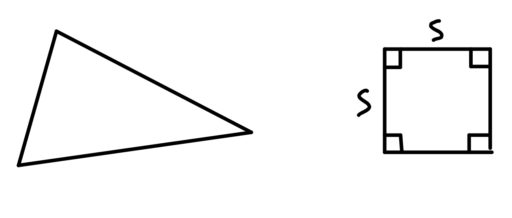Then you can conclude that the triangle is to the left of the square. Or if you see: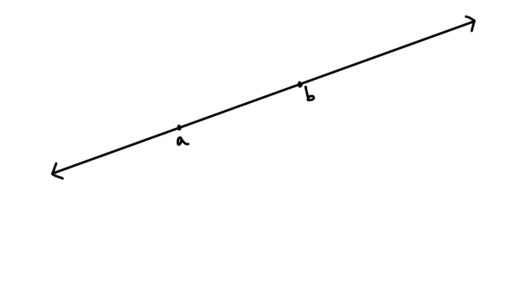You can conclude that those two points are on that line, and that point a is to the left of point b.

Finally, the rules are different for co-ordinate systems and the number line:

Rule 3: Co-ordinate systems and the number line are to scale.

The main co-ordinate system you will see on the GRE is the xy-plane: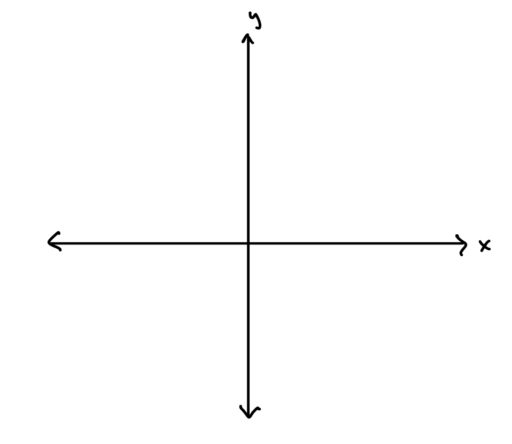and the number line is just the following diagram: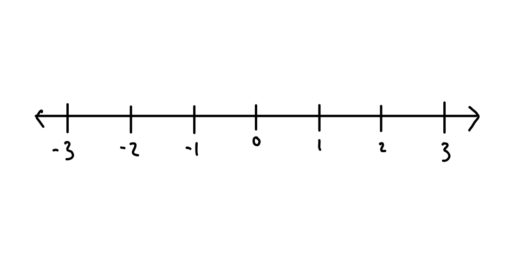On such systems, the objects are to scale. So if you see: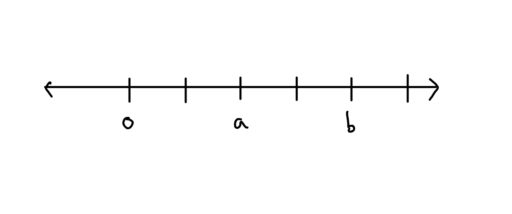you can conclude that the value foris twice as large as the value forOr if you see: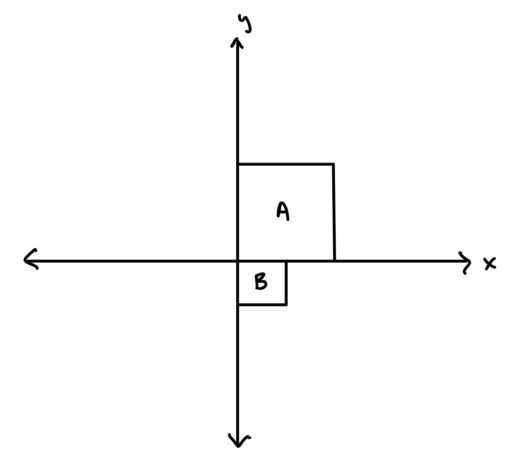you can conclude that the area of the smaller square is 1/4 of the area of the larger square.

So in short, remember that the proportions of shapes cannot be trusted, but the relative position of shapes can be trusted, and if there is co-ordinate system or a number line, then even the proportions can be trusted.